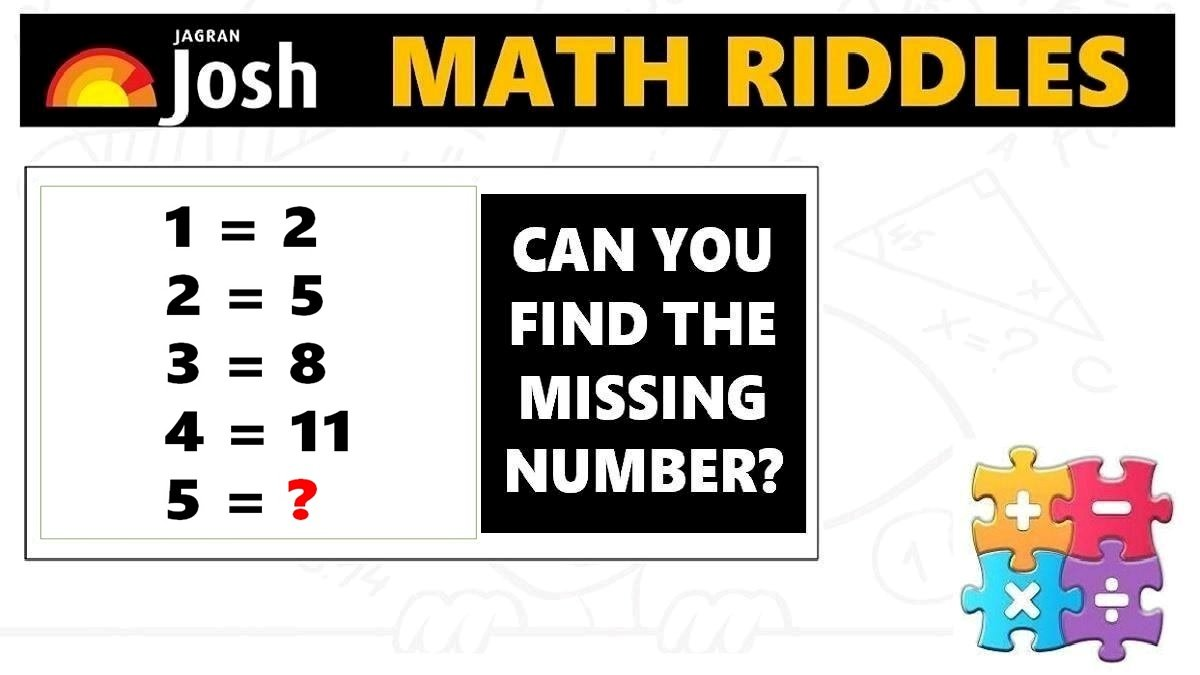# Math Riddles with Answers: Logic Puzzles That Will Teach Fast Maths Tricks

Math Riddles Challenge: Do you want to learn fast maths tricks to solve logic puzzles? Are you preparing for competitive exams? Check out these math riddles!Math Riddles with Answers: Logic Puzzles That Will Teach Fast Maths Tricks

Math Riddles: If you are looking to crack reasoning and quantitative aptitude sections in competitive exams then you should definitely learn these fast maths tricks. Below, we have two math riddles for which you have to find the missing numbers. First, you will have to figure out the logic and apply the tricks to find the answers. But hey, remember the challenge is to solve them in less than 1 minute each.

Explanation:

Explanation:

## Tell us in comments: Did you solve these mathematics puzzles in less than 1 minute each?

Check out more math puzzles!